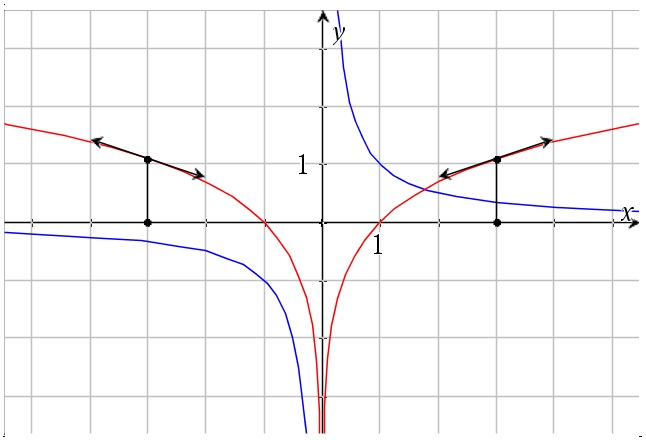# Some standard integrals

Up a level : Integrals
Previous page : The connection between the definite and indefinite integral
Next page : Integration by substitutionWe can get a number of integrals by basically use the differential rules backwards. We have that$\int {f(x)dx = F(x) + C}$

and that$\frac{d}{{dx}}F(x) = f(x)$

From this we get

 Rule number: f(x) F(x) 1 u(x)±v(x) u(x) ±v(x) 2 cu(x) cU(x) 3 c cx 4${x^n}$${x^n}$$\frac{{{x^{n + 1}}}}{{n + 1}},\quad n \ne - 1$$\frac{{{x^{n + 1}}}}{{n + 1}},\quad n \ne - 1$ 5 1/x ln|x| 6 ex ex 7 sin x −cos x 8 cos x sin x

Remember that the above table gives us the indefinite integral i.e. the primitive function without the constant of integration and that a function written with capital letters is a primitive function of the corresponding function written with small letters.

1. The first two we might call meta-rules. They are rules about integrable functions in general. The first one states that if we have two functions plus or minus each other then we can integrate them separately.  That rules follows immediately from the corresponding differential rule, as does all of these. Example:$\int {({x^2} + x - {e^x})dx = } \frac{{{x^3}}}{3} + \frac{{{x^2}}}{2} - {e^x} + C$

As you can see this rule holds even if we have more than two terms. You can easily check the above result by differentiating the left hand side.

1. If we have a constant factor, then we just keep it. Yet again it simply the same as the corresponding differential rule.$\begin{gathered} \int {(3{x^2} + 5x - 7{e^x})dx = } 3 \cdot \frac{{{x^3}}}{3} + 5 \cdot \frac{{{x^2}}}{2} - 7{e^x} + C \hfill \\ \quad = {x^3} + \frac{5}{2}{x^2} - 7{e^x} + C \hfill \\ \end{gathered}$

1. This is just an example of the above, since a primitive function of 1 is x.
2. You can check this by taking the derivative of what we got:${\left( {\frac{{{x^{n + 1}}}}{{n + 1}}} \right)^\prime } = {\left( {\frac{1}{{n + 1}}{x^{n + 1}}} \right)^\prime } = \frac{{n + 1}}{{n + 1}}{x^{n + 1 - 1}} = {x^n}$

1. Here we know that the derivative of ln x is 1/x, but why the absolute value on the indefinite integral? Just to remind you, the absolute value of a real number x, |x|=the distance to the origin, i.e. the positive number corresponding to the distance to the origin (that way of thinking of the absolute value will help you when it comes to absolute values of say complex numbers). We thus have |5|=|-5|=5.

Let us look at say x=3 and x=−3. Since the slope of ln x when x is positive is 1/x, then,  of reasons of symmetry, the slope of ln(-x) when x is negative must be the same, but with opposite sign.Another way to see this: The function ln|x| must be an even function, i.e. a function symmetric about the y-axis, and since the mirror images slopes in the opposite directions then the derivative must have the opposite sign, just as 1/x has, and since 1/x is the derivative of ln x as x is positive, then therefore the derivative of 1/x as x is negative is ln(−x) and we thus get that the derivative of ln|x| is 1/x and thus that the primitive function of 1/x is ln|x|.

The rest is rather straight forward.Up a level : Integrals
Previous page : The connection between the definite and indefinite integral
Next page : Integration by substitutionLast modified: Feb 21, 2022 @ 14:57